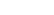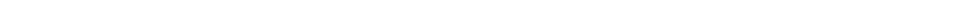Problem 11
Q

# Particles of charge $+65$, $+48$, and $-95 \; \mu \textrm{C}$ and are placed in a line (Fig. 16–52). The center one is 0.35 m from each of the others. Calculate the net force on each charge due to the other two.Figure 16-52.A
$F_1 = -1.2 \times 10^2 \textrm{ N, }$$F_2 = 5.6 \times 10^2 \textrm{ N, }$$F_3 = -4.5 \times 10^2 \textrm{ N}$By moonpen on Wed, 3/23/2016 - 6:47 PM

when calculating the Force of 2, why isn't the force from 2 to 1 negative?

By Mr. Dychko on Thu, 3/24/2016 - 2:46 AM

Hi moopen, thanks for the question. When calculating the net force on charge 2 we're interested only in forces exerted on charge 2. The charge 1 is exerting a force to the right on charge 2, so that force is taken as positive. It's true that charge 2 exerts a force to the left on charge 1 (this is the Newton's 3rd Law counterpart to the force exerted on charge 2 by charge 1), but this force isn't relevant since it isn't exerted on charge 2.

Hope that helps,
Mr. Dychko

By acw2085 on Sat, 1/15/2022 - 5:27 PM

It seems more intuitive to mark pushes as positive because of how the book defines attractive force as negative and positive force as repelling. Is there some other thing behind this seeming contradictory technique- for instance, does it prepare us for something later on doing it this way? Or, am I misunderstanding something?

Giancoli Answers, including solutions and videos, is copyright © 2009-2023 Shaun Dychko, Vancouver, BC, Canada. Giancoli Answers is not affiliated with the textbook publisher. Book covers, titles, and author names appear for reference purposes only and are the property of their respective owners. Giancoli Answers is your best source for the 7th and 6th edition Giancoli physics solutions.